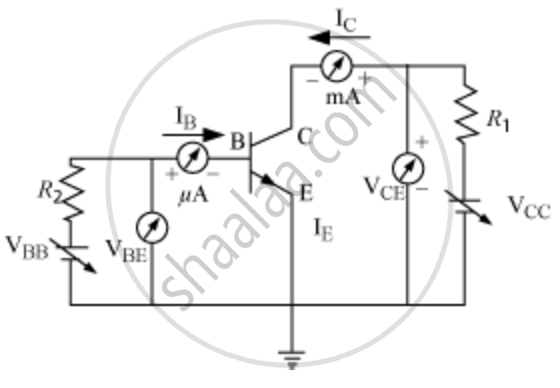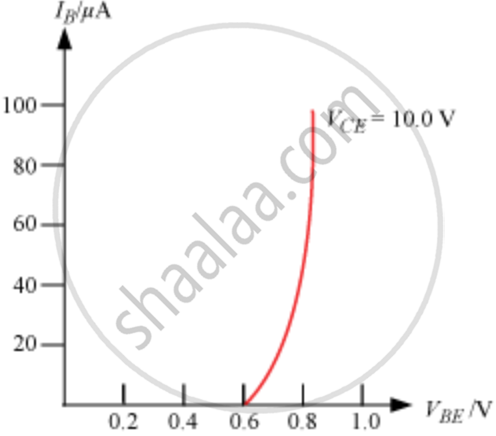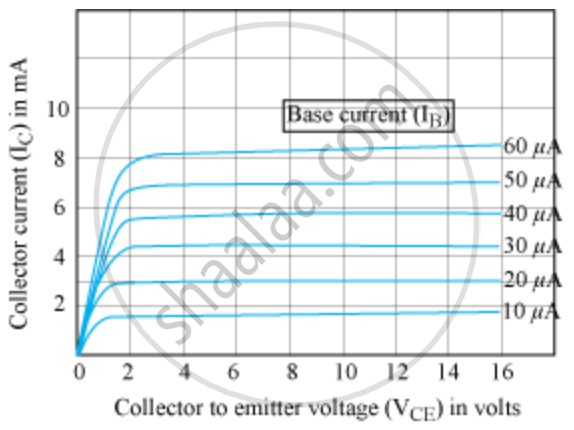Share

# Draw a Typical Input and Output Characteristics of an N-p-n Transistor in Ce Configuration. Show How These Characteristics Can Be Used to Determine (A) the Input Resistance (R1), and (B) Current Amplification Factor (β) - CBSE (Science) Class 12 - Physics

ConceptTransistor and Characteristics of a Transistor

#### Question

Draw a typical input and output characteristics of an n-p-n transistor in CE configuration. Show how these characteristics can be used to determine (a) the input resistance (r1), and (b) current amplification factor (β)

#### Solution

CE configuration is the most widely used transistor.Variation of base current (IB) with the base-emitter voltage VBE is called input characteristics.

Variation of collector current IC with the collector − Emitter voltage VCE is called output characteristics

Input characteristicsOutput characteristicsParameters of Transistors

Input resistance (ri)

r_i = ((triangleV_"BE")/(triangleI_B))_(V_"CE")

ΔVBE − Base-emitter voltage

ΔIB − Base current

VCE − Constant collector-emitter voltage

Output resistance (r0)

r_0 = ((triangleV_"CE")/(triangleI_C))_(I_B)

ΔVCE − Collector-emitter voltage

ΔIC − Collector current

IB − Base current

Current amplification factor (β)

beta_(ac) = ((triangleI_c)/(triangleI_B))_(V_(CE))

IC − Collector current

IB − Base current

VCE − Constant collector-emitter voltage.

Is there an error in this question or solution?

#### Video TutorialsVIEW ALL 

Solution Draw a Typical Input and Output Characteristics of an N-p-n Transistor in Ce Configuration. Show How These Characteristics Can Be Used to Determine (A) the Input Resistance (R1), and (B) Current Amplification Factor (β) Concept: Transistor and Characteristics of a Transistor.
S